Click on the snowman to donate now!

It’s here! Brrrrr!
A chill wind does chide!
But never fear…
Arrived at Northside!
(Don’t let this chance slide…)
Imagine!
A short opportunity
A tax-deductible donation from you
Will keep our school’s budget sunny!

Five generous donor families are waiting for your dollars to match, up to a total of \$30,000. The deadline for the challenge is January 9, 2019.

# Why Donate to Northside?

Friends of Northside provides critical funding to our school that helps ensure unparalleled educational standards, along with extracurricular activities that matter to our students.

Your gift helps offset the \$500,000 funding deficit. The school needs everyone's support to maintain staffing, activities, and more.

If you have not donated and are able, to please consider giving. Every \$ counts.

As the end of the year approaches, remember:

▪ All donations are 100% tax deductible.
▪ Gifts of stock are accepted and can be an effective tax-planning strategy.
▪ Employer donor-matching programs can double or triple the impact of your gift.
 ▪ All donations are 100% tax deductible.
 ▪ Gifts of stock are accepted and can be an effective tax-planning strategy.
 ▪ Employer donor-matching programs can double or triple the impact of your gift.

## Please watch these short videos to see reasons why your donations are so important.

 table.module-5{width:78.3%;padding:0}table div table+table+table+table+table+table div table{width:78.3%;float:none;margin-left:auto;margin-right:auto;padding:0}table div table+table+table+table+table+table div table a{border:0 none;text-decoration:none}table div table+table+table+table+table+table div table img{width:100%!important;border:0 none;text-decoration:none}table div table+table+table+table+table+table div table td{width:100%;padding:0}/* styles */
 table div table+table+table+table+table+table+table+table div table{width:100%;padding:0}table div table+table+table+table+table+table+table+table div table img{width:96.23%;padding:0;float:none}table div table+table+table+table+table+table+table+table div table td{width:100%;padding:0 1.88% 18px}/* styles */# PARENTS, WE NEED YOUR HELP!

## Teacher and Staff Appreciation Holiday Luncheon Buffet

Thursday, December 20

Our teachers and staff are THE BEST, so let's show them how grateful we are for all they do for our students! Contributions of homemade or purchased lunch and dessert items, drinks, gift cards, financial support, and your time are all welcome.

Please consider signing up to contribute to the Faculty and Staff Holiday Luncheon. There are a lot of spots still open in the SignUp. Click HERE to sign up.

## Holiday Luncheon Committee Co-Chair Needed

The Holiday Luncheon Committee is looking for a Co-Chair to help with the event this year and take over next year. It's one of the Faculty and Staff's favorite days. If you are interested or have questions please contact Nancy Liljedahl at n.liljedahl@comcast.net.

 table div table+table+table+table+table+table+table+table+table+table div table{width:100%;padding:0}table div table+table+table+table+table+table+table+table+table+table div table img{width:96.23%;padding:0;float:none}table div table+table+table+table+table+table+table+table+table+table div table td{width:100%;padding:0 1.88% 18px}/* styles */# Class of 2019: Gap Year Program Scholarship with Global Citizen Year

To win 1 of 10 full scholarships for CPS graduating seniors to join Global Citizen Year in 2019-2020, contact your counselor by Thursday, December 20th.

Global Citizen Year is a non-profit gap year program on a mission to create a pipeline of global leaders with the insight, skills, and conviction to build a better world. Each year Global Citizen Year recruits a diverse group of 150+ high school graduates with strong leadership potential and supports them through a school-year long immersion in communities across Latin America, Asia, and Africa. During their Global Citizen Year, Fellows live with a local family, apprentice with a community organization, and become fluent in a foreign language. The organization has country offices in its four locations (Brazil, Ecuador, India, and Senegal) and local staff to support and mentor Fellows throughout the 8-month immersion experience.

 table div table+table+table+table+table+table+table+table+table+table+table+table div table{width:100%;padding:0}table div table+table+table+table+table+table+table+table+table+table+table+table div table img{width:96.23%;padding:0;float:none}table div table+table+table+table+table+table+table+table+table+table+table+table div table td{width:100%;padding:0 1.88% 18px}/* styles */# This Week in Sports

Come to a game and support our student athletes!

▪ Monday, December 17 at 5:30 pm (SO) and 7:00 pm (V) vs. Christian Liberty Academy at Christian Liberty Academy
▪ Tuesday, December 18, 5:00 pm (V) and 6:30 pm (SO) vs. Sullivan HS at NCP
▪ Saturday, December 22 at 1:00 pm (V) vs. Elmwood Park at NCP
 ▪ Monday, December 17 at 5:30 pm (SO) and 7:00 pm (V) vs. Christian Liberty Academy at Christian Liberty Academy
 ▪ Tuesday, December 18, 5:00 pm (V) and 6:30 pm (SO) vs. Sullivan HS at NCP
 ▪ Saturday, December 22 at 1:00 pm (V) vs. Elmwood Park at NCP

▪ Wednesday, December 19 at 5:30 pm (SO) and 7:00 pm (V) vs. Yeshiva at NCP
▪ Saturday, December 22 at 6:00 pm (F) vs. Juarez HS at Quest Multi-Sport
 ▪ Wednesday, December 19 at 5:30 pm (SO) and 7:00 pm (V) vs. Yeshiva at NCP
 ▪ Saturday, December 22 at 6:00 pm (F) vs. Juarez HS at Quest Multi-Sport

## Men's Bowling

▪ Monday, December 17 at 4:15 pm vs. Schurz HS at Home (Waveland Bowl)
 ▪ Monday, December 17 at 4:15 pm vs. Schurz HS at Home (Waveland Bowl)

## Women’s Bowling

▪ Monday, December 17 at 4:15 pm vs. Westinghouse at Home (Waveland Bowl)
 ▪ Monday, December 17 at 4:15 pm vs. Westinghouse at Home (Waveland Bowl)

## Men’s Swimming and Diving

Practices began on Monday, November 19. Email Coach Ceja for more information.

## Men’s & Women’s Indoor Track & Field

Indoor season will begin on Monday, January 14. Contact Director of Athletics Henderson for details.

## Wrestling

The wrestling team will co-op with Von Steuben High School this year. Contact Director of Athletics Henderson for details.

 table div table+table+table+table+table+table+table+table+table+table+table+table+table+table+table div table{width:100%;padding:0}table div table+table+table+table+table+table+table+table+table+table+table+table+table+table+table div table img{width:96.23%;padding:0;float:none}table div table+table+table+table+table+table+table+table+table+table+table+table+table+table+table div table td{width:100%;padding:0 1.88% 18px}/* styles */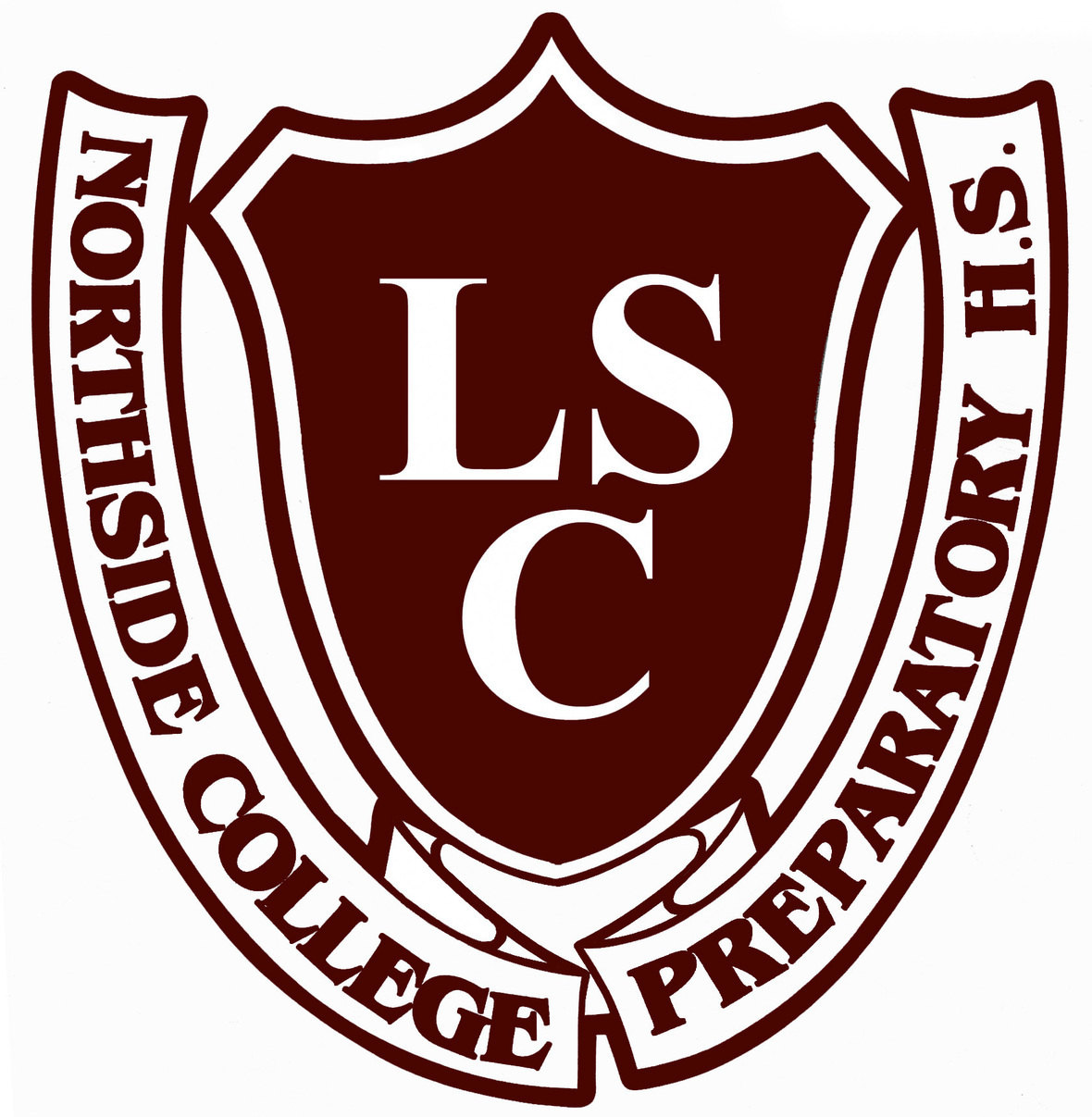# LSC Meeting

Tuesday, December 18
6:30 pm
Faculty Lounge

 table div table+table+table+table+table+table+table+table+table+table+table+table+table+table+table+table+table div table{width:100%;padding:0}table div table+table+table+table+table+table+table+table+table+table+table+table+table+table+table+table+table div table img{width:96.23%;padding:0;float:none}table div table+table+table+table+table+table+table+table+table+table+table+table+table+table+table+table+table div table td{width:100%;padding:0 1.88% 18px}/* styles */# Cozy Concert

Tuesday, December 18
7:00 pm

 table div table+table+table+table+table+table+table+table+table+table+table+table+table+table+table+table+table+table+table div table{width:100%;padding:0}table div table+table+table+table+table+table+table+table+table+table+table+table+table+table+table+table+table+table+table div table img{width:96.23%;padding:0;float:none}table div table+table+table+table+table+table+table+table+table+table+table+table+table+table+table+table+table+table+table div table td{width:100%;padding:0 1.88% 18px}/* styles */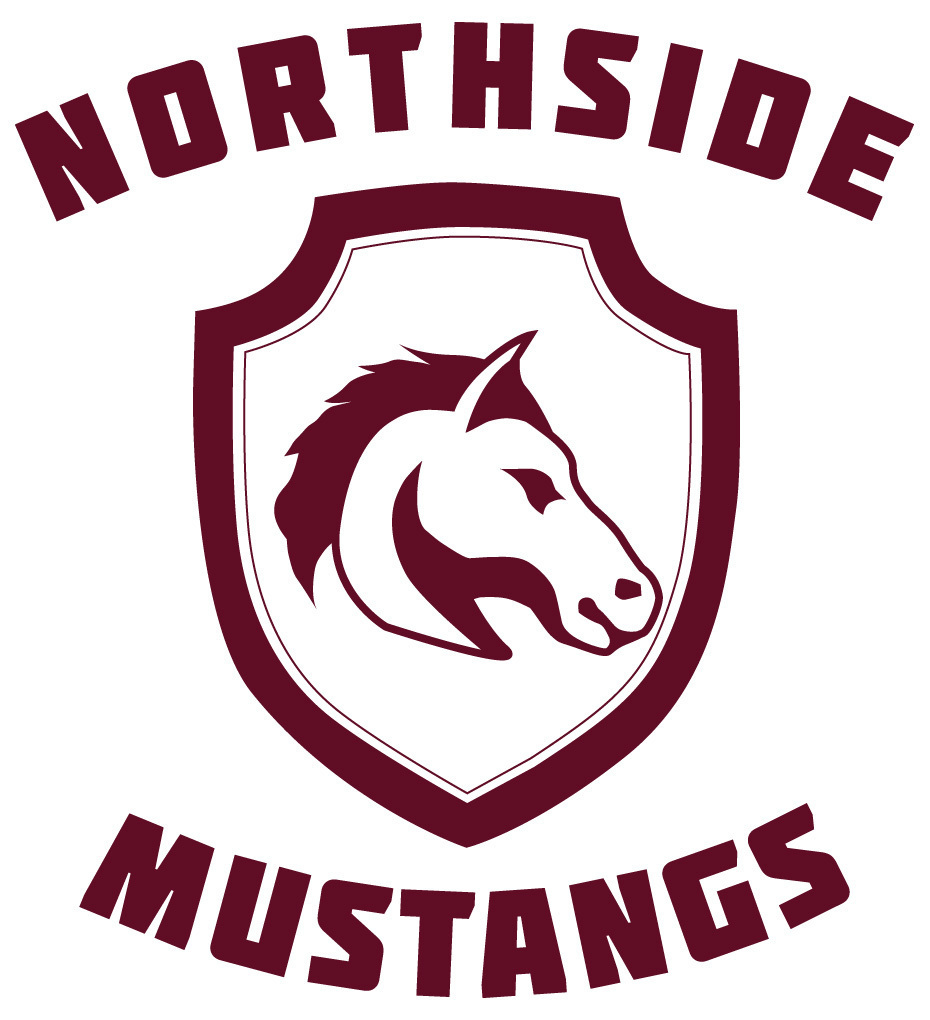Pick up NCP swag for the special students on your gift list.

Tuesday, December 18
7:30 - 7:55 am and 3:15 - 3:45 pm

Wednesday, December 19
7:30 - 7:55 am and 3:15 - 3:45 pm

Thursday, December 20
7:30 - 7:55 am and 3:15 - 3:45 pm

 table div table+table+table+table+table+table+table+table+table+table+table+table+table+table+table+table+table+table+table+table+table div table{width:100%;padding:0}table div table+table+table+table+table+table+table+table+table+table+table+table+table+table+table+table+table+table+table+table+table div table img{width:96.23%;padding:0;float:none}table div table+table+table+table+table+table+table+table+table+table+table+table+table+table+table+table+table+table+table+table+table div table td{width:100%;padding:0 1.88% 18px}/* styles */# REGISTER FOR AP EXAMS

Students who plan to take an Advanced Placement (AP) exam this spring must register and pay by 5:00 am, December 28.

The cost is \$110 per exam. Click HERE to register online and pay using a credit or debit card.

 table div table+table+table+table+table+table+table+table+table+table+table+table+table+table+table+table+table+table+table+table+table+table+table div table{width:100%;padding:0}table div table+table+table+table+table+table+table+table+table+table+table+table+table+table+table+table+table+table+table+table+table+table+table div table img{width:96.23%;padding:0;float:none}table div table+table+table+table+table+table+table+table+table+table+table+table+table+table+table+table+table+table+table+table+table+table+table div table td{width:100%;padding:0 1.88% 18px}/* styles */# Doing Your Holiday Shopping Online?

## You can help our school in the process, with no extra cost to you.

AmazonSmile is a simple and automatic way to support Northside while you shop.

To choose Northside, please note that our AmazonSmile name is Friends of "North" "Side" (North Side - two words at AmazonSmile).

 table div table+table+table+table+table+table+table+table+table+table+table+table+table+table+table+table+table+table+table+table+table+table+table+table+table+table div table{width:100%;padding:0}table div table+table+table+table+table+table+table+table+table+table+table+table+table+table+table+table+table+table+table+table+table+table+table+table+table+table div table img{width:96.23%;padding:0;float:none}table div table+table+table+table+table+table+table+table+table+table+table+table+table+table+table+table+table+table+table+table+table+table+table+table+table+table div table td{width:100%;padding:0 1.88% 18px}/* styles */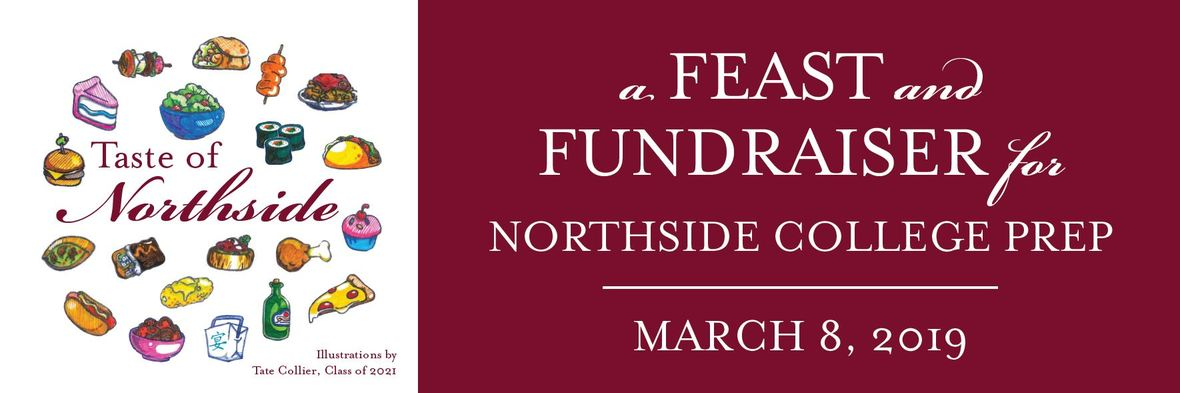# 2019 Spring Benefit Update## Action Items Needed

The solicitation committee needs your help to secure more items for the live and silent auctions – sports tickets, restaurant gift cards, travel and hospitality vouchers, tutoring, unique experiences, hosted parent parties, etc. Please email Kristen Werries Collier, kristenwerriescollier@gmail.com, to donate, get more information, or pass on connections to pursue. Click HERE for the donation form.Corporate Sponsorship contributions will be used to underwrite the cost of the 2019 Spring Benefit and enable Friends of Northside to provide more of its financial resources for programs and services for the school not covered by the CPS budget. For sponsorship opportunities and in-kind donations, please contact Matt Schechter, matthew.d.schechter@gmail.com. Click HERE for the sponsorship form.We are putting together a slideshow of our students participating in the many activities that make Northside special. If you have pictures you'd like to be considered for inclusion in the presentation, please forward them to Debra Swan at debra@theswans.net.

## Food & Beverages Needed

Have a connection to a local restaurant or beverage vendor? We need items to add to our dinner buffet, as well as wine, champagne, and soda. Contact Debra Swan, debra@theswans.net, if you can help.

 table div table+table+table+table+table+table+table+table+table+table+table+table+table+table+table+table+table+table+table+table+table+table+table+table+table+table+table+table+table+table+table+table+table div table{width:100%;padding:0}table div table+table+table+table+table+table+table+table+table+table+table+table+table+table+table+table+table+table+table+table+table+table+table+table+table+table+table+table+table+table+table+table+table div table img{width:96.23%;padding:0;float:none}table div table+table+table+table+table+table+table+table+table+table+table+table+table+table+table+table+table+table+table+table+table+table+table+table+table+table+table+table+table+table+table+table+table div table td{width:100%;padding:0 1.88% 18px}/* styles */The deadline for purchasing the 2018-2019 Stampede for the standard price of \$45.00 is Friday, March 1. After this date the price will go up from \$45.00 to \$55.00. Also, after this date, you won’t be guaranteed a yearbook, since we will not be ordering many extra copies.

2) Enter code 4187219

 table div table+table+table+table+table+table+table+table+table+table+table+table+table+table+table+table+table+table+table+table+table+table+table+table+table+table+table+table+table+table+table+table+table+table+table div table{width:100%;padding:0}table div table+table+table+table+table+table+table+table+table+table+table+table+table+table+table+table+table+table+table+table+table+table+table+table+table+table+table+table+table+table+table+table+table+table+table div table img{width:96.23%;padding:0;float:none}table div table+table+table+table+table+table+table+table+table+table+table+table+table+table+table+table+table+table+table+table+table+table+table+table+table+table+table+table+table+table+table+table+table+table+table div table td{width:100%;padding:0 1.88% 18px}/* styles */# Homecoming Dance

## Chaperones Needed

Friday, January 18
7:00 - 10:00 pm

 table div table+table+table+table+table+table+table+table+table+table+table+table+table+table+table+table+table+table+table+table+table+table+table+table+table+table+table+table+table+table+table+table+table+table+table+table+table div table{width:100%;padding:0}table div table+table+table+table+table+table+table+table+table+table+table+table+table+table+table+table+table+table+table+table+table+table+table+table+table+table+table+table+table+table+table+table+table+table+table+table+table div table img{width:96.23%;padding:0;float:none}table div table+table+table+table+table+table+table+table+table+table+table+table+table+table+table+table+table+table+table+table+table+table+table+table+table+table+table+table+table+table+table+table+table+table+table+table+table div table td{width:100%;padding:0 1.88% 18px}/* styles */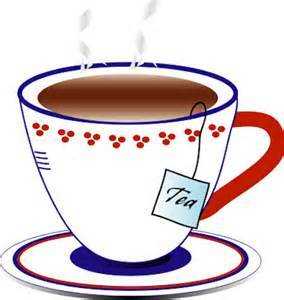# Donate Tea to the Faculty Lounge

## Individually Wrapped Tea Bags Needed

Many members of our faculty and staff drink tea, and the supply in the faculty lounge is running low. Please consider donating a few boxes of individually wrapped tea bags to NCP. Mark your package FOR FON and drop it off in the main office or at the security desk.

 table div table+table+table+table+table+table+table+table+table+table+table+table+table+table+table+table+table+table+table+table+table+table+table+table+table+table+table+table+table+table+table+table+table+table+table+table+table+table+table div table{width:100%;padding:0}table div table+table+table+table+table+table+table+table+table+table+table+table+table+table+table+table+table+table+table+table+table+table+table+table+table+table+table+table+table+table+table+table+table+table+table+table+table+table+table div table img{width:96.23%;padding:0;float:none}table div table+table+table+table+table+table+table+table+table+table+table+table+table+table+table+table+table+table+table+table+table+table+table+table+table+table+table+table+table+table+table+table+table+table+table+table+table+table+table div table td{width:100%;padding:0 1.88% 18px}/* styles */Ms. Woodruff is hoping to run a sewing colloquium this spring but needs sewing machines. If you have a sewing machine you can donate, please contact Ms. Woodruff at jwoodruff@cps.edu.

 table div table+table+table+table+table+table+table+table+table+table+table+table+table+table+table+table+table+table+table+table+table+table+table+table+table+table+table+table+table+table+table+table+table+table+table+table+table+table+table+table+table div table{width:100%;padding:0}table div table+table+table+table+table+table+table+table+table+table+table+table+table+table+table+table+table+table+table+table+table+table+table+table+table+table+table+table+table+table+table+table+table+table+table+table+table+table+table+table+table div table img{width:96.23%;padding:0;float:none}table div table+table+table+table+table+table+table+table+table+table+table+table+table+table+table+table+table+table+table+table+table+table+table+table+table+table+table+table+table+table+table+table+table+table+table+table+table+table+table+table+table div table td{width:100%;padding:0 1.88% 18px}/* styles */# Travel this Spring with Northside

## Spring Break 2019 Trip to Spain

Four amazing cities and more than 15 sites to visit, including Madrid, Granada, Sevilla, and Costa del Sol!

What is included? 8 days of beautiful and historical España! Airfare, all hotel stays, transportation-comfortable coach bus, tour guides, breakfast and dinner, etc.

Open to ALL students.

## Spring Break 2019 Trip to England

On this trip you will experience the distinct character that is London. Admire St Paul's Cathedral, Big Ben,The House of Parliament, and Buckingham Palace. We will also travel to Somerset and visit Stonehenge the United Kingdom's most mysterious landmark, Bath, Stratford (see where William Shakespeare was born), and Oxford.

See Ms. Fernandez or Ms. Vosnos or click HERE for an informational brochure.

## Spring Break 2019 Trip to the Grand Canyon

Students will have a chance to connect with the majesty of our National Park system. We will spend 5 nights in the Grand Canyon camping, hiking, stargazing, and learning wilderness skills. Students will participate in a second-semester colloquium to prepare them for the trip. Trip sponsors and chaperones include Ms. Qazi, Ms. Schaldenbrand, and Mr. Barry Smith. Approximate cost: \$1,000 (includes flight, food, and lodging).

 table div table+table+table+table+table+table+table+table+table+table+table+table+table+table+table+table+table+table+table+table+table+table+table+table+table+table+table+table+table+table+table+table+table+table+table+table+table+table+table+table+table+table+table div table{width:100%;padding:0}table div table+table+table+table+table+table+table+table+table+table+table+table+table+table+table+table+table+table+table+table+table+table+table+table+table+table+table+table+table+table+table+table+table+table+table+table+table+table+table+table+table+table+table div table img{width:96.23%;padding:0;float:none}table div table+table+table+table+table+table+table+table+table+table+table+table+table+table+table+table+table+table+table+table+table+table+table+table+table+table+table+table+table+table+table+table+table+table+table+table+table+table+table+table+table+table+table div table td{width:100%;padding:0 1.88% 18px}/* styles */# Parking Lot Safety and Procedures

Our NCP school parking lot is a busy place before and after school. Families dropping off and picking up students are asked to be mindful of both safety and courtesy. Please assist us by ensuring that traffic lanes stay clear during drop-off and pick-up times. Pulling over to the curb on the right as your student enters or exits your vehicle keeps students safe and traffic flow efficient.

Please remember that ALL VEHICLES MUST EXIT ONTO KEDZIE. You may enter the parking lot from Kedzie or Bryn Mawr, but regardless of where you enter, all cars must exit onto Kedzie. For the safety of our students and the rest of the Northside community, please do not exit onto Bryn Mawr.

 table div table+table+table+table+table+table+table+table+table+table+table+table+table+table+table+table+table+table+table+table+table+table+table+table+table+table+table+table+table+table+table+table+table+table+table+table+table+table+table+table+table+table+table+table+table+table div table{width:100%;padding:0}table div table+table+table+table+table+table+table+table+table+table+table+table+table+table+table+table+table+table+table+table+table+table+table+table+table+table+table+table+table+table+table+table+table+table+table+table+table+table+table+table+table+table+table+table+table+table div table img{width:96.23%;padding:0;float:none}table div table+table+table+table+table+table+table+table+table+table+table+table+table+table+table+table+table+table+table+table+table+table+table+table+table+table+table+table+table+table+table+table+table+table+table+table+table+table+table+table+table+table+table+table+table+table div table td{width:100%;padding:0 1.88% 18px}/* styles */# Winter Break

## No School

December 24 - January 4
Classes resume Monday, January 7, 2019.

## Winter Break for Us, Too!

This Week at Northside will be on Winter Break and return Sunday, January 6, 2019.

Friends of Northside wishes you and your family a restful, peaceful, and wonderful Winter Break.

Happy New Year!

 table div table+table+table+table+table+table+table+table+table+table+table+table+table+table+table+table+table+table+table+table+table+table+table+table+table+table+table+table+table+table+table+table+table+table+table+table+table+table+table+table+table+table+table+table+table+table+table+table div table{width:100%;padding:0}table div table+table+table+table+table+table+table+table+table+table+table+table+table+table+table+table+table+table+table+table+table+table+table+table+table+table+table+table+table+table+table+table+table+table+table+table+table+table+table+table+table+table+table+table+table+table+table+table div table img{width:96.23%;padding:0;float:none}table div table+table+table+table+table+table+table+table+table+table+table+table+table+table+table+table+table+table+table+table+table+table+table+table+table+table+table+table+table+table+table+table+table+table+table+table+table+table+table+table+table+table+table+table+table+table+table+table div table td{width:100%;padding:0 1.88% 18px}/* styles */# January Dates to Note

January 11, 8:00 am: Friends of Northside First Friday
January 11, 7:00 pm: Dance Showcase
January 15, 6:30 pm: LSC Meeting
January 18, 7:00 pm: Homecoming Dance
January 21: Martin Luther King Jr. Day (No School)
January 22, 7:00 pm: Friends of Northside Meeting
January 28 - 31: First Semester Final Exams

 table div table+table+table+table+table+table+table+table+table+table+table+table+table+table+table+table+table+table+table+table+table+table+table+table+table+table+table+table+table+table+table+table+table+table+table+table+table+table+table+table+table+table+table+table+table+table+table+table+table+table div table{width:100%;padding:0}table div table+table+table+table+table+table+table+table+table+table+table+table+table+table+table+table+table+table+table+table+table+table+table+table+table+table+table+table+table+table+table+table+table+table+table+table+table+table+table+table+table+table+table+table+table+table+table+table+table+table div table img{width:96.23%;padding:0;float:none}table div table+table+table+table+table+table+table+table+table+table+table+table+table+table+table+table+table+table+table+table+table+table+table+table+table+table+table+table+table+table+table+table+table+table+table+table+table+table+table+table+table+table+table+table+table+table+table+table+table+table div table td{width:100%;padding:0 1.88% 18px}/* styles */# CPS Volunteer Requirements

According to CPS policy, anybody who is going to be in contact with students must be approved as a CPS volunteer. There are two levels of volunteers, Level 1 and Level 2.

## Level II Volunteers

(This process can be completed in a day & is required even of volunteers for Friends of Northside events)
A fingerprint criminal background check is not required for Level II Volunteers.

A Level II Volunteer is:

1. A parent providing supervised volunteer service in their child’s school or classroom for less than 10 hours/week;
2. Any individual providing volunteer service for less than 5 hours/week at a school where s/he does not have a child enrolled;
3. An individual providing incidental volunteer service with no ongoing individualized interaction with a student(s) including those who speak at a class/assembly, judge academic competitions, give musical performance, participate in the “Principal for a Day” program, job shadowing event or other one-time event provided where there is direct supervision of the activity/event by regular school employees;
4. A parent accompanying his/her child’s class on a one-day field trip or another type of extracurricular activity that does not involve an overnight stay; and/or
5. An individual providing volunteer service on projects/activities involving no or nominal contact with children (home-based volunteer activities, volunteers serving at Central or Network office).
 1 A parent providing supervised volunteer service in their child’s school or classroom for less than 10 hours/week;
 2 Any individual providing volunteer service for less than 5 hours/week at a school where s/he does not have a child enrolled;
 3 An individual providing incidental volunteer service with no ongoing individualized interaction with a student(s) including those who speak at a class/assembly, judge academic competitions, give musical performance, participate in the “Principal for a Day” program, job shadowing event or other one-time event provided where there is direct supervision of the activity/event by regular school employees;
 4 A parent accompanying his/her child’s class on a one-day field trip or another type of extracurricular activity that does not involve an overnight stay; and/or
 5 An individual providing volunteer service on projects/activities involving no or nominal contact with children (home-based volunteer activities, volunteers serving at Central or Network office).

## Level I Volunteers

(Please allow 8-12 weeks for the entire process)
A fingerprint criminal background check is required for all Level I Volunteers.

A Level I volunteer is:

1. A parent providing volunteer service in their child’s school or classroom for 10 or more hours/week;
2. An individual providing volunteer service for 5 or more hours/week at a school where s/he does not have a child enrolled; and/or
3. Any individual chaperoning an overnight school-sponsored trip, regardless of the hours/week that the volunteer serves (See also the Student Travel Policy).
 1 A parent providing volunteer service in their child’s school or classroom for 10 or more hours/week;
 2 An individual providing volunteer service for 5 or more hours/week at a school where s/he does not have a child enrolled; and/or
 3 Any individual chaperoning an overnight school-sponsored trip, regardless of the hours/week that the volunteer serves (See also the Student Travel Policy).

To become a volunteer, please visit CPS' Volunteer website and follow the instructions. There is also a link on Northside's website on the Parents page Northside Volunteers.

Assistant Principal Kaitlin O'Hare is Northside's Volunteer Coordinator. After applying to become a volunteer, you must visit the school and show Ms. O'Hare your ID, so she can approve you to move forward in the volunteer process. For Level II volunteers, that is the only step you must take. For Level I, you will receive further instructions from CPS.

 table div table+table+table+table+table+table+table+table+table+table+table+table+table+table+table+table+table+table+table+table+table+table+table+table+table+table+table+table+table+table+table+table+table+table+table+table+table+table+table+table+table+table+table+table+table+table+table+table+table+table+table+table div table{width:100%;padding:0}table div table+table+table+table+table+table+table+table+table+table+table+table+table+table+table+table+table+table+table+table+table+table+table+table+table+table+table+table+table+table+table+table+table+table+table+table+table+table+table+table+table+table+table+table+table+table+table+table+table+table+table+table div table img{width:96.23%;padding:0;float:none}table div table+table+table+table+table+table+table+table+table+table+table+table+table+table+table+table+table+table+table+table+table+table+table+table+table+table+table+table+table+table+table+table+table+table+table+table+table+table+table+table+table+table+table+table+table+table+table+table+table+table+table+table div table td{width:100%;padding:0 1.88% 18px}/* styles */table.module-52{width:94.34%;padding:0}table div table+table+table+table+table+table+table+table+table+table+table+table+table+table+table+table+table+table+table+table+table+table+table+table+table+table+table+table+table+table+table+table+table+table+table+table+table+table+table+table+table+table+table+table+table+table+table+table+table+table+table+table+table div table{width:94.34%;float:none;margin-left:auto;margin-right:auto;padding:0}table div table+table+table+table+table+table+table+table+table+table+table+table+table+table+table+table+table+table+table+table+table+table+table+table+table+table+table+table+table+table+table+table+table+table+table+table+table+table+table+table+table+table+table+table+table+table+table+table+table+table+table+table+table div table a{border:0 none;text-decoration:none}table div table+table+table+table+table+table+table+table+table+table+table+table+table+table+table+table+table+table+table+table+table+table+table+table+table+table+table+table+table+table+table+table+table+table+table+table+table+table+table+table+table+table+table+table+table+table+table+table+table+table+table+table+table div table img{width:100%!important;border:0 none;text-decoration:none}table div table+table+table+table+table+table+table+table+table+table+table+table+table+table+table+table+table+table+table+table+table+table+table+table+table+table+table+table+table+table+table+table+table+table+table+table+table+table+table+table+table+table+table+table+table+table+table+table+table+table+table+table+table div table td{width:100%;padding:0}/* styles */
 /* styles */ Check out The HoofBeat, Northside's online newspaper, to get NCP news from the student perspective!
 table div table+table+table+table+table+table+table+table+table+table+table+table+table+table+table+table+table+table+table+table+table+table+table+table+table+table+table+table+table+table+table+table+table+table+table+table+table+table+table+table+table+table+table+table+table+table+table+table+table+table+table+table+table+table+table div table{width:100%;padding:0}table div table+table+table+table+table+table+table+table+table+table+table+table+table+table+table+table+table+table+table+table+table+table+table+table+table+table+table+table+table+table+table+table+table+table+table+table+table+table+table+table+table+table+table+table+table+table+table+table+table+table+table+table+table+table+table div table img{width:96.23%;padding:0;float:none}table div table+table+table+table+table+table+table+table+table+table+table+table+table+table+table+table+table+table+table+table+table+table+table+table+table+table+table+table+table+table+table+table+table+table+table+table+table+table+table+table+table+table+table+table+table+table+table+table+table+table+table+table+table+table+table div table td{width:100%;padding:0 1.88% 18px}/* styles */table.module-55{width:57.17%;padding:0}table div table+table+table+table+table+table+table+table+table+table+table+table+table+table+table+table+table+table+table+table+table+table+table+table+table+table+table+table+table+table+table+table+table+table+table+table+table+table+table+table+table+table+table+table+table+table+table+table+table+table+table+table+table+table+table+table div table{width:57.17%;float:none;margin-left:auto;margin-right:auto;padding:0}table div table+table+table+table+table+table+table+table+table+table+table+table+table+table+table+table+table+table+table+table+table+table+table+table+table+table+table+table+table+table+table+table+table+table+table+table+table+table+table+table+table+table+table+table+table+table+table+table+table+table+table+table+table+table+table+table div table a{border:0 none;text-decoration:none}table div table+table+table+table+table+table+table+table+table+table+table+table+table+table+table+table+table+table+table+table+table+table+table+table+table+table+table+table+table+table+table+table+table+table+table+table+table+table+table+table+table+table+table+table+table+table+table+table+table+table+table+table+table+table+table+table div table img{width:100%!important;border:0 none;text-decoration:none}table div table+table+table+table+table+table+table+table+table+table+table+table+table+table+table+table+table+table+table+table+table+table+table+table+table+table+table+table+table+table+table+table+table+table+table+table+table+table+table+table+table+table+table+table+table+table+table+table+table+table+table+table+table+table+table+table div table td{width:100%;padding:0}/* styles */

# What is FAN?

FAN is Family Action Network, an organization which connects parents, educators, and professionals through collaborative programming that educates, inspires, and positively impacts the broader community. Nearly every week during the school year there will be a dynamic and important speaker, discussing the issues that interest you.

FAN events are free to all.

# Frederick Douglass: Prophet of Freedom

David Blight, Ph.D.
Class of 1954 Professor of American History and Director of the Gilder Lehrman Center for the Study of Slavery, Resistance, and Abolition, Yale University.
Recipient of the Bancroft Prize, the Abraham Lincoln Prize, and the Frederick Douglass Prize, among others.
Author or editor of a dozen books, including American Oracle: The Civil War in the Civil Rights Era; Race and Reunion: The Civil War in American Memory; and the brand-new Frederick Douglass: Prophet of Freedom
Friday, Jan. 25, 2019
7:00 pm
Evanston Township High School Auditorium
1600 Dodge Ave., Evanston

# Out of Many Faiths: Religious Diversity and the American Promise

Eboo Patel
Founder and President of Interfaith Youth Core
Wednesday, Jan. 30, 2019
7:00 pm
Evanston Township High School Auditorium
1600 Dodge Ave., Evanston

 table div table+table+table+table+table+table+table+table+table+table+table+table+table+table+table+table+table+table+table+table+table+table+table+table+table+table+table+table+table+table+table+table+table+table+table+table+table+table+table+table+table+table+table+table+table+table+table+table+table+table+table+table+table+table+table+table+table+table div table{width:100%;padding:0}table div table+table+table+table+table+table+table+table+table+table+table+table+table+table+table+table+table+table+table+table+table+table+table+table+table+table+table+table+table+table+table+table+table+table+table+table+table+table+table+table+table+table+table+table+table+table+table+table+table+table+table+table+table+table+table+table+table+table div table img{width:96.23%;padding:0;float:none}table div table+table+table+table+table+table+table+table+table+table+table+table+table+table+table+table+table+table+table+table+table+table+table+table+table+table+table+table+table+table+table+table+table+table+table+table+table+table+table+table+table+table+table+table+table+table+table+table+table+table+table+table+table+table+table+table+table+table div table td{width:100%;padding:0 1.88% 18px}/* styles */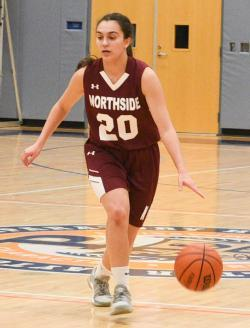# Alina Yamin ('19) Reaches 2,000 Point Milestone

Senior varsity basketball player Alina Yamin ('19) became the first girl and only the second player ever in the history of Northside College Prep to score 2,000 points in their career at the school.

Yamin joins Northside alumn, Robert Eschmann ('05) as the only players to achieve the 2,000 point milestone.

# Congratulations to Northside's Poms Team

Northside’s Poms team earned 3rd place out of 10 competitive dance teams at the IHSA Waubonsie Valley Competition this past weekend. Congrats to the team for their hard work and thank you to the staff for your support!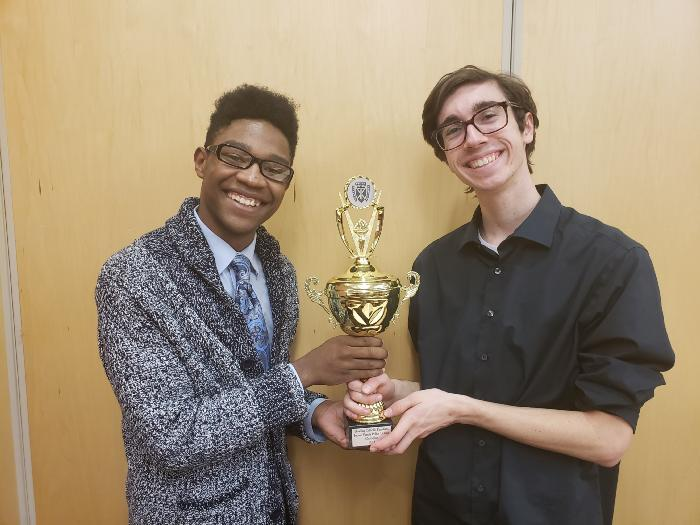# NCP Debate has Big Success at Dowling Catholic Paradigm Tournament

Varsity Debate team members, Kathy Martinez (20th overall speaker) and Wasim Rahaman (11th overall speaker) received their first bid to the national Tournament of Champions making it all the way through to the semifinals to become eligible for the national championship tournament in the spring.

Magi Ortiz (8th overall speaker) and Alex Pinheiro (7th overall speaker) picked up their fourth TOC bid (leading the state of Illinois) on a 3-0 decision over Lane Tech.

On the JV team, sophomores Caleb DeBerry and Ben Katz won the tournament championship on a 2-1 decision over Glenbrook South.

 table div table+table+table+table+table+table+table+table+table+table+table+table+table+table+table+table+table+table+table+table+table+table+table+table+table+table+table+table+table+table+table+table+table+table+table+table+table+table+table+table+table+table+table+table+table+table+table+table+table+table+table+table+table+table+table+table+table+table+table+table+table+table+table div table{width:100%;padding:0}table div table+table+table+table+table+table+table+table+table+table+table+table+table+table+table+table+table+table+table+table+table+table+table+table+table+table+table+table+table+table+table+table+table+table+table+table+table+table+table+table+table+table+table+table+table+table+table+table+table+table+table+table+table+table+table+table+table+table+table+table+table+table+table div table img{width:96.23%;padding:0;float:none}table div table+table+table+table+table+table+table+table+table+table+table+table+table+table+table+table+table+table+table+table+table+table+table+table+table+table+table+table+table+table+table+table+table+table+table+table+table+table+table+table+table+table+table+table+table+table+table+table+table+table+table+table+table+table+table+table+table+table+table+table+table+table+table div table td{width:100%;padding:0 1.88% 18px}/* styles */# FON Connect

Friends of Northside uses a customer/constituent relationship management (CRM) system known as FON Connect that allows our parent volunteer organizations to work from one database. It is not a CPS database and is completely different and separate from the CPS Parent Portal and Northside's EPay website.

FON Connect serves as the NCP Family Directory and allows users to review their donation history, update their contact information, register for upcoming events, and more. Each parent has a separate account. Transactions, including donations, are recorded in the account of the parent who initiated the transaction or donation.

If you’re new to FON Connect, you'll need to set up a login and password. To do so:

 table div table+table+table+table+table+table+table+table+table+table+table+table+table+table+table+table+table+table+table+table+table+table+table+table+table+table+table+table+table+table+table+table+table+table+table+table+table+table+table+table+table+table+table+table+table+table+table+table+table+table+table+table+table+table+table+table+table+table+table+table+table+table+table+table+table div table{width:100%;padding:0}table div table+table+table+table+table+table+table+table+table+table+table+table+table+table+table+table+table+table+table+table+table+table+table+table+table+table+table+table+table+table+table+table+table+table+table+table+table+table+table+table+table+table+table+table+table+table+table+table+table+table+table+table+table+table+table+table+table+table+table+table+table+table+table+table+table div table img{width:96.23%;padding:0;float:none}table div table+table+table+table+table+table+table+table+table+table+table+table+table+table+table+table+table+table+table+table+table+table+table+table+table+table+table+table+table+table+table+table+table+table+table+table+table+table+table+table+table+table+table+table+table+table+table+table+table+table+table+table+table+table+table+table+table+table+table+table+table+table+table+table+table div table td{width:100%;padding:0 1.88% 18px}/* styles */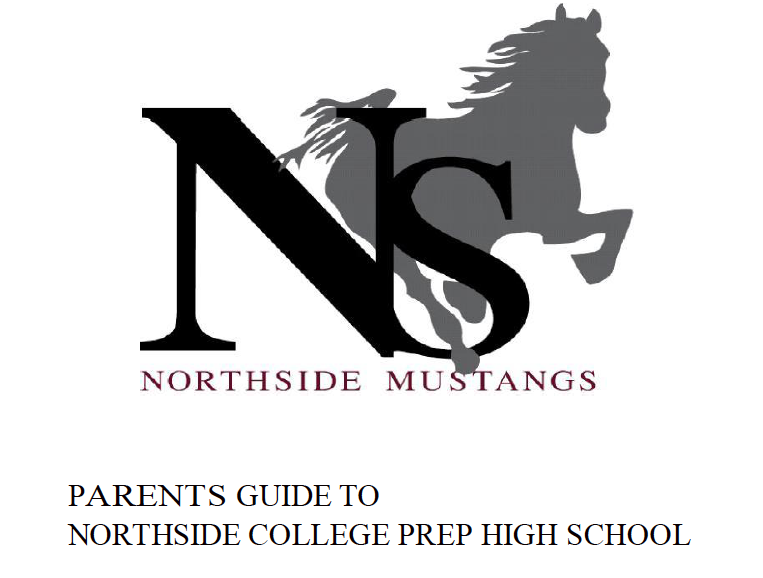# Navigating Northside

Navigating Northside, our parent handbook, is available on the school website under the Parents tab. Click HERE to access it directly.

 table div table+table+table+table+table+table+table+table+table+table+table+table+table+table+table+table+table+table+table+table+table+table+table+table+table+table+table+table+table+table+table+table+table+table+table+table+table+table+table+table+table+table+table+table+table+table+table+table+table+table+table+table+table+table+table+table+table+table+table+table+table+table+table+table+table+table+table div table{width:100%;padding:0}table div table+table+table+table+table+table+table+table+table+table+table+table+table+table+table+table+table+table+table+table+table+table+table+table+table+table+table+table+table+table+table+table+table+table+table+table+table+table+table+table+table+table+table+table+table+table+table+table+table+table+table+table+table+table+table+table+table+table+table+table+table+table+table+table+table+table+table div table img{width:96.23%;padding:0;float:none}table div table+table+table+table+table+table+table+table+table+table+table+table+table+table+table+table+table+table+table+table+table+table+table+table+table+table+table+table+table+table+table+table+table+table+table+table+table+table+table+table+table+table+table+table+table+table+table+table+table+table+table+table+table+table+table+table+table+table+table+table+table+table+table+table+table+table+table div table td{width:100%;padding:0 1.88% 18px}/* styles */# Upcoming College Visits at Northside

Thursday, December 20
Peace Corps Vanuatu, 3:15 pm

The list of college visits is updated frequently. For the most current information, parents of juniors and seniors should log into their parent account for Naviance.

 table div table+table+table+table+table+table+table+table+table+table+table+table+table+table+table+table+table+table+table+table+table+table+table+table+table+table+table+table+table+table+table+table+table+table+table+table+table+table+table+table+table+table+table+table+table+table+table+table+table+table+table+table+table+table+table+table+table+table+table+table+table+table+table+table+table+table+table+table+table+table+table div table{width:100%;padding:0}table div table+table+table+table+table+table+table+table+table+table+table+table+table+table+table+table+table+table+table+table+table+table+table+table+table+table+table+table+table+table+table+table+table+table+table+table+table+table+table+table+table+table+table+table+table+table+table+table+table+table+table+table+table+table+table+table+table+table+table+table+table+table+table+table+table+table+table+table+table+table+table div table img{width:96.23%;padding:0;float:none}table div table+table+table+table+table+table+table+table+table+table+table+table+table+table+table+table+table+table+table+table+table+table+table+table+table+table+table+table+table+table+table+table+table+table+table+table+table+table+table+table+table+table+table+table+table+table+table+table+table+table+table+table+table+table+table+table+table+table+table+table+table+table+table+table+table+table+table+table+table+table+table div table td{width:100%;padding:0 1.88% 18px}/* styles */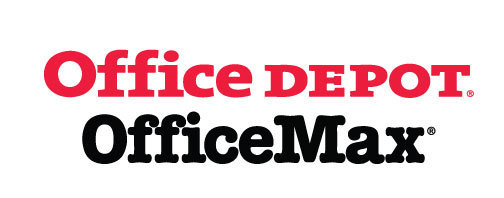# Give Back to Northside

Purchase school and office supplies at Office Depot and OfficeMax, and Northside can receive 5% back in credits or FREE supplies. Simply provide our school ID 70031171 at checkout, online or in stores. There’s no cost to you!

 table div table+table+table+table+table+table+table+table+table+table+table+table+table+table+table+table+table+table+table+table+table+table+table+table+table+table+table+table+table+table+table+table+table+table+table+table+table+table+table+table+table+table+table+table+table+table+table+table+table+table+table+table+table+table+table+table+table+table+table+table+table+table+table+table+table+table+table+table+table+table+table+table+table+table div table{width:100%;padding:0}table div table+table+table+table+table+table+table+table+table+table+table+table+table+table+table+table+table+table+table+table+table+table+table+table+table+table+table+table+table+table+table+table+table+table+table+table+table+table+table+table+table+table+table+table+table+table+table+table+table+table+table+table+table+table+table+table+table+table+table+table+table+table+table+table+table+table+table+table+table+table+table+table+table+table div table img{width:96.23%;padding:0;float:none}table div table+table+table+table+table+table+table+table+table+table+table+table+table+table+table+table+table+table+table+table+table+table+table+table+table+table+table+table+table+table+table+table+table+table+table+table+table+table+table+table+table+table+table+table+table+table+table+table+table+table+table+table+table+table+table+table+table+table+table+table+table+table+table+table+table+table+table+table+table+table+table+table+table+table div table td{width:100%;padding:0 1.88% 18px}/* styles */# Follow Us on Social Media

▪ Northside Prep Parents' Facebook Page, a closed group for parents, by parents, to share their knowledge of Northside
▪ Friends of Northside Facebook Page, for news everyone can view
 ▪ Northside Prep Parents' Facebook Page, a closed group for parents, by parents, to share their knowledge of Northside
 ▪ Friends of Northside Facebook Page, for news everyone can view
 table div table+table+table+table+table+table+table+table+table+table+table+table+table+table+table+table+table+table+table+table+table+table+table+table+table+table+table+table+table+table+table+table+table+table+table+table+table+table+table+table+table+table+table+table+table+table+table+table+table+table+table+table+table+table+table+table+table+table+table+table+table+table+table+table+table+table+table+table+table+table+table+table+table+table+table+table div table{width:100%;padding:0}table div table+table+table+table+table+table+table+table+table+table+table+table+table+table+table+table+table+table+table+table+table+table+table+table+table+table+table+table+table+table+table+table+table+table+table+table+table+table+table+table+table+table+table+table+table+table+table+table+table+table+table+table+table+table+table+table+table+table+table+table+table+table+table+table+table+table+table+table+table+table+table+table+table+table+table+table div table img{width:96.23%;padding:0;float:none}table div table+table+table+table+table+table+table+table+table+table+table+table+table+table+table+table+table+table+table+table+table+table+table+table+table+table+table+table+table+table+table+table+table+table+table+table+table+table+table+table+table+table+table+table+table+table+table+table+table+table+table+table+table+table+table+table+table+table+table+table+table+table+table+table+table+table+table+table+table+table+table+table+table+table+table+table div table td{width:100%;padding:0 1.88% 18px}/* styles */Northside’s website can be found HERE.

Friends of Northside's website can be found HERE.

 table div table+table+table+table+table+table+table+table+table+table+table+table+table+table+table+table+table+table+table+table+table+table+table+table+table+table+table+table+table+table+table+table+table+table+table+table+table+table+table+table+table+table+table+table+table+table+table+table+table+table+table+table+table+table+table+table+table+table+table+table+table+table+table+table+table+table+table+table+table+table+table+table+table+table+table+table+table+table div table{width:100%;padding:0}table div table+table+table+table+table+table+table+table+table+table+table+table+table+table+table+table+table+table+table+table+table+table+table+table+table+table+table+table+table+table+table+table+table+table+table+table+table+table+table+table+table+table+table+table+table+table+table+table+table+table+table+table+table+table+table+table+table+table+table+table+table+table+table+table+table+table+table+table+table+table+table+table+table+table+table+table+table+table div table img{width:96.23%;padding:0;float:none}table div table+table+table+table+table+table+table+table+table+table+table+table+table+table+table+table+table+table+table+table+table+table+table+table+table+table+table+table+table+table+table+table+table+table+table+table+table+table+table+table+table+table+table+table+table+table+table+table+table+table+table+table+table+table+table+table+table+table+table+table+table+table+table+table+table+table+table+table+table+table+table+table+table+table+table+table+table+table div table td{width:100%;padding:0 1.88% 18px}/* styles */